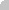Specifies whether the Mask property masks a floating point number.Type Description Boolean A boolean expression that indicates whether the Mask property masks a floating point number.

If the MaskFloat property is True, the Mask property may indicate the followings:

• negative number: if the first character in the mask is - ( minus ) the control supports negative numbers. Pressing the - key will toggle the sign of the number. The + sign is never displayed.
• decimal symbol: the last character that's different than # ( digit ), or 0 (zero) indicates the decimal symbol. If it is not present the control mask a floating point number without decimals.
• thousand symbol: the thousand symbol is the last character that's not a # ( digit ), 0 (zero) or it is not the decimal symbol as explained earlier, if present.
• the maximum number of decimals in the number ( the # or 0 character after the decimal symbol )
• the maximum number of digits in the integer part ( the number of # or 0 character before decimal symbol )
• the 0 character indicates a leading-zero. The count of 0 (zero) characters before decimal character indicates the leading-zero for integer part of the control, while the count of 0 (zero) characters after the decimal separator indicates the leading-zero for decimal part of the control. For instance, the Mask on "-###,###,##0.00",  while the control's Text property is 1, the control displays 1.00, if 1.1 if displays 1.10, and if empty, the 0.00 is displayed.

If the Mask property is empty, the control takes the settings for the regional options like: Decimal Symbol , No. of digits after decimal, Digit grouping symbol.

Here are few samples:

The mask "-###.###.##0,00" filter floating point numbers a number for German settings ( "," is the decimal sign, "." is the thousands separator ). This format displays leading-zeros.

The mask "-###.###.###,##" filter floating point numbers a number for German settings ( "," is the decimal sign, "." is the thousands separator )

The mask "-###,###,###.##" filter floating point numbers a number for English settings ( "." is the decimal sign, "," is the thousands separator )

The mask "####" indicates a max-4 digit number ( positive ) without a decimal symbol and without digit grouping

The mask "-##.#" filters a floating point number from the -99.9 to 99.9 ( "." is the decimal sign,  no thousands separator )

The mask "#,###.##" filters a floating point number from the 0 to 9,999.99 with digit grouping ( "." is the decimal sign, "," is the thousands separator ).

Send comments on this topic.